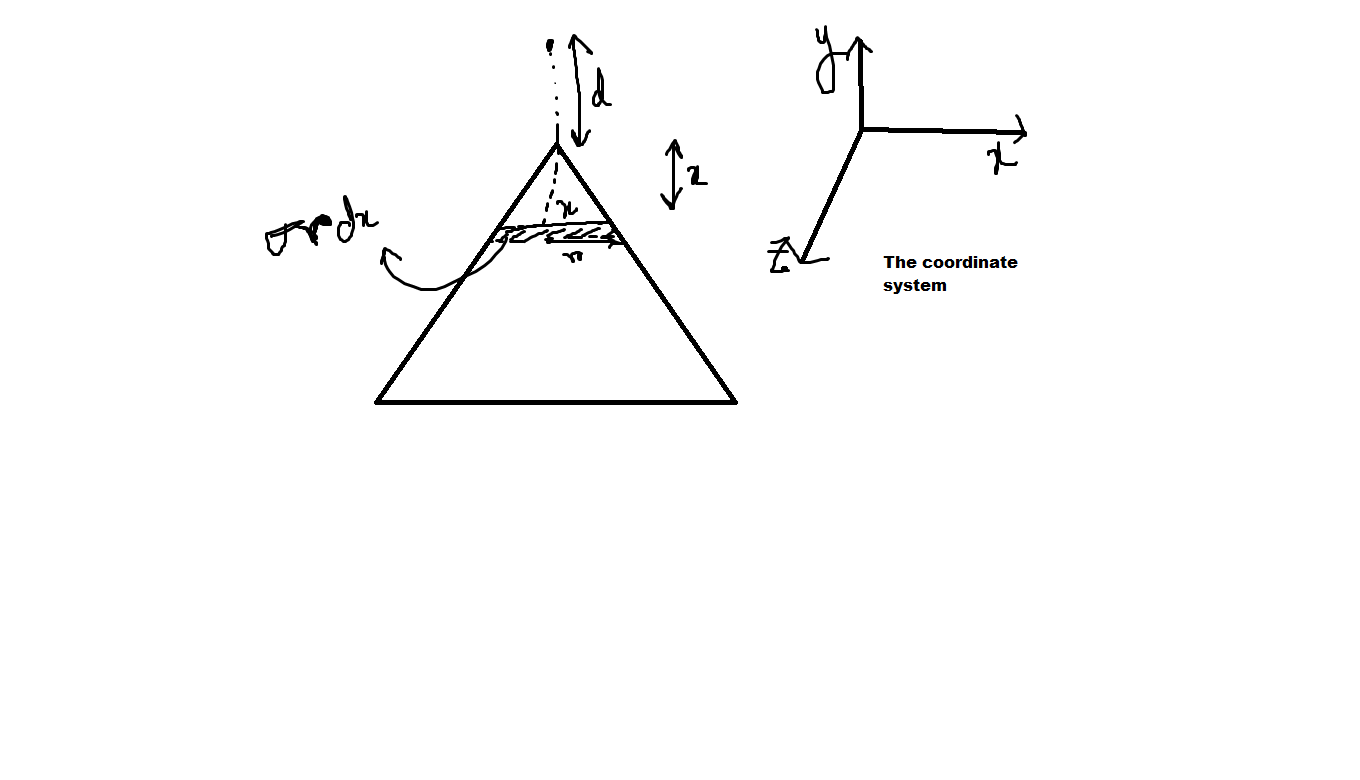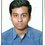# Doubt on Ronak's Question.

https://brilliant.org/problems/electric-fields-due-to-different-kinds-of-plates/.

The link is given.

Here, while doing with Integration results in different value. I will be glad if someone points out where i am wrong.

QUESTION

We have a triangular uniformly charged plate of charge density $\sigma$.

We take a point just above a vertex of the triangular plate at a distance $d$ perpendicular to the plane of the triangle. Let the vertical component of electric field at that point be $E(d)$ in $V/m$

Find $\displaystyle \lim_{d \rightarrow 0}{E(d)}$

Details and Assumptions:

1) $\sigma = 10^{-9} C , {\epsilon}_{0}=8.85 \times {10}^{-12} \text{ SI units}$

2) The triangle is equilateral. By vertical component of electric field I mean electric field perpendicular to the plane of charge.

3) The plate is non conducting.

MY SOLUTIONSo By using Proportionality in triangle,

We get

$\dfrac{r}{x} = \dfrac{\dfrac{a}{2}}{\dfrac{\sqrt{3}a}{2}}$

$\implies r= \dfrac{x}{\sqrt{3}}$ .

Now for electric field, We have,

$dE \sin\theta = \dfrac{kd (dq)}{(x+d)^{\tiny \dfrac{3}{2}}}$

$E_{y} = \displaystyle{\int_{0}^{\sqrt{3}a/2} \dfrac{kd (dq)}{(x+d)^{\tiny \dfrac{3}{2}}}}$

We also know $dq= \sigma r dx$.

After solving, we get $\displaystyle{\lim_{d \rightarrow 0} E(d)= 10.39}$.

If I am wrong anywhere, Please help me correct it. Also the integral calculation can be a error.

Thanks for looking through my doubt.Note by Md Zuhair
3 years, 2 months ago

This discussion board is a place to discuss our Daily Challenges and the math and science related to those challenges. Explanations are more than just a solution — they should explain the steps and thinking strategies that you used to obtain the solution. Comments should further the discussion of math and science.

When posting on Brilliant:

• Use the emojis to react to an explanation, whether you're congratulating a job well done , or just really confused .
• Ask specific questions about the challenge or the steps in somebody's explanation. Well-posed questions can add a lot to the discussion, but posting "I don't understand!" doesn't help anyone.
• Try to contribute something new to the discussion, whether it is an extension, generalization or other idea related to the challenge.
• Stay on topic — we're all here to learn more about math and science, not to hear about your favorite get-rich-quick scheme or current world events.

MarkdownAppears as
*italics* or _italics_ italics
**bold** or __bold__ bold
- bulleted- list
• bulleted
• list
1. numbered2. list
1. numbered
2. list
Note: you must add a full line of space before and after lists for them to show up correctly
paragraph 1paragraph 2

paragraph 1

paragraph 2

[example link](https://brilliant.org)example link
> This is a quote
This is a quote
    # I indented these lines
# 4 spaces, and now they show
# up as a code block.

print "hello world"
# I indented these lines
# 4 spaces, and now they show
# up as a code block.

print "hello world"
MathAppears as
Remember to wrap math in $$ ... $$ or $ ... $ to ensure proper formatting.
2 \times 3 $2 \times 3$
2^{34} $2^{34}$
a_{i-1} $a_{i-1}$
\frac{2}{3} $\frac{2}{3}$
\sqrt{2} $\sqrt{2}$
\sum_{i=1}^3 $\sum_{i=1}^3$
\sin \theta $\sin \theta$
\boxed{123} $\boxed{123}$

## Comments

Sort by:

Top Newest

Please help me! I may have skipped some names. You can add them too!

- 3 years, 2 months ago

Log in to reply

I just solved it by integrating, with no simplifying tricks like the one given in the solution (ingenious as it is). I'll put up a solution in the next few days.

- 3 years, 2 months ago

Log in to reply

That means my integral is correct, But the value isnt?

- 3 years, 2 months ago

Log in to reply

I used an integral, but it wasn't necessarily your integral.

- 3 years, 2 months ago

Log in to reply

Ohkay, I see. I dont know why, I am not getting it. Did you just saw my solution. You can point out any mistake if you get there. else it may be fine.

- 3 years, 2 months ago

Log in to reply

When calculating the contribution from one of the strips, you have to integrate as well. So the problem effectively boils down to a double integral. But I did the two integrations sequentially.

- 3 years, 2 months ago

Log in to reply

Okay, So I have to take a small element in that strip too?

- 3 years, 2 months ago

Log in to reply

Yes. So integrating with infinitesimals within the strip gives you an expression for the contribution of a strip. Then integrating the contributions from the strips gives the final result. The first integral is readily done with an integral table. For the second integral, I used Wolfram Alpha. Then you have some interesting philosophizing to do regarding the limit of an arctangent before getting to the final answer.

- 3 years, 2 months ago

Log in to reply

Okay, Will try in that way! Thanks!

- 3 years, 2 months ago

Log in to reply

Thanks sir! Finally solved it using Integrals! But, Why do i need double integral here? Why cant i apply integral from the middle of the thin strip in the triangle?

- 3 years, 2 months ago

Log in to reply

Did you do it by integrating the contributions from the strips?

- 3 years, 2 months ago

Log in to reply

Yes sir. Like the way you told

- 3 years, 2 months ago

Log in to reply

Ok. And then it also takes an integral to figure out the contribution from a strip. That's why I refer to the whole process as effectively being a double integral.

- 3 years, 2 months ago

Log in to reply

Your way of writing fields due to an infinitismal element is wrong.Use a related result of finite length charged wire.$E=\frac {k\lambda }{d}(\sin \theta _{1}+\sin \theta _{2})$ along the radial direction and$\frac {k\lambda }{d}(\cos \theta _{1}-\cos \theta _{2})$ along the perpendicular. But if you want to develop your skills avoid integrals,symmetry helps a no of times,like considering hexagonal plates.

- 3 years, 2 months ago

Log in to reply

I have a small qs. If does that $\dfrac{\sigma}{2 \epsilon_{0}}$ can be used for Any kind of plates? As i knew it is used for rectangular plates. Thanks

- 3 years, 2 months ago

Log in to reply

In this Q the concept of relative largeness plays a very important role. d->0 indirectly states that the point is near an infinitely large plane. I'll check if we can arrive at the same conclusion using the integration process.

- 3 years, 2 months ago

Log in to reply

×

Problem Loading...

Note Loading...

Set Loading...